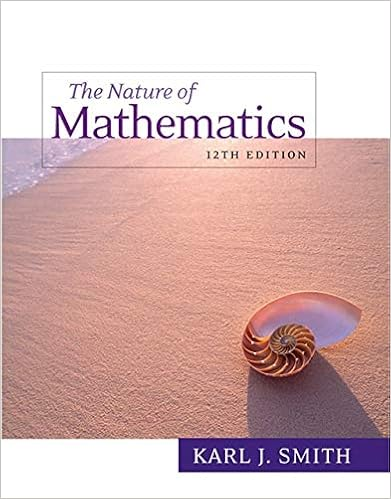# B a qq t for some matrix q with orthonormal columns u

• Notes
• 56

This preview shows page 27 - 29 out of 56 pages.

##### We have textbook solutions for you!
The document you are viewing contains questions related to this textbook.The document you are viewing contains questions related to this textbook.
Chapter 4 / Exercise 51
Nature of Mathematics
SmithExpert Verified
b. A = QQ T , for some matrix Q with orthonormal columns u 1 , . . . , u m . Note that Q T Q = I m , since the ij th entry of Q T Q is u i · u j . Then A 2 = QQ T QQ T = Q ( Q T Q ) Q T = QI m Q T = QQ T = A . 43. Examine how A acts on u , and on a vector v orthogonal to u : Au = (2 uu T - I 3 ) u = 2 uu T u - u = u, since u T u = u · u = u 2 = 1 . Av = (2 uu T - I 3 ) v = 2 uu T v - v = - v, since u T v = u · v = 0 . Since A leaves the vectors in L = span( u ) unchanged and reverses the vectors in V = L , it represents the reflection about L . Note that B = - A , so that B reverses the vectors in L and leaves the vectors in V unchanged; that is, B represents the reflection about V . 44. Note that A T is an m × n matrix. By Facts 3.3.7 and 5.3.9c we have dim(ker( A T )) = n - rank( A T ) = n - rank( A ). By Fact 3.3.6, dim(im( A )) = rank( A ), so that dim(im( A )) + dim(ker( A T )) = n . 45. Note that A T is an m × n matrix. By Facts 3.3.7 and 5.3.9c, we have dim(ker( A )) = m - rank( A ) and dim(ker( A T )) = n - rank( A T ) = n - rank( A ), so that dim(ker( A )) = dim(ker( A T )) if (and only if) A is a square matrix. 260
##### We have textbook solutions for you!
The document you are viewing contains questions related to this textbook.The document you are viewing contains questions related to this textbook.
Chapter 4 / Exercise 51
Nature of Mathematics
SmithExpert Verified
ISM: Linear Algebra Section 5.3 46. By Fact 5.2.2, the columns u 1 , . . . , u m of Q are orthonormal. Therefore, Q T Q = I m , since the ij th entry of Q T Q is u i · u j . If we multiply the equation M = QR by Q T from the left then Q T M = Q T QR = R , as claimed. 47. By Fact 5.2.2, the columns u 1 , . . . , u m of Q are orthonormal. Therefore, Q T Q = I m , since the ij th entry of Q T Q is u i · u j . By Fact 5.3.9a, we now have A T A = ( QR ) T QR = R T Q T QR = R T R . 48. As suggested, we consider the QR factorization A T = PR of A T , where P is orthogonal and R is upper triangular with positive diagonal entries. By Fact 5.3.9a, A = ( PR ) T = R T P T . Note that L = R T is lower triangular and Q = P T is orthogonal. 49. Yes! By Exercise 5.2.45, we can write A T = PL , where P is orthogonal and L is lower triangular. By Fact 5.3.9a, A = ( PL ) T = L T P T . Note that R = L T is upper triangular, and Q = P T is orthogonal (by Exercise 11). 50. a. If an n × n matrix A is orthogonal and upper triangular, then A - 1 is both lower triangular (since A - 1 = A T ) and upper triangular (being the inverse of an upper triangular matrix; compare with Exercise 2.3.35c). Therefore, A - 1 = A T is a diagonal matrix, and so is A itself. Since A is orthogonal with positive diagonal entries, all the diagonal entries must be 1, so that A = I n . b. Using the terminology suggested in the hint, we observe that 51. a. Using the terminology suggested in the hint, we observe that I m = Q T 1 Q 1 = ( Q 2 S ) T Q 2 S = S T Q T 2 Q 2 S = S T S , so that S is orthogonal, by Fact 5.3.7. b. Using the terminology suggested in the hint, we observe that R 2 R - 1 1 is both orthogonal (let S = R 2 R - 1 1 in part a) and upper triangular, with positive diagonal entries. By Exercise 50a, we have R 2 R - 1 1 = I m , so that R 1 = R 2 . Then Q 1 = Q 2 R 2 R - 1 1 = Q 2 , as claimed.
•••